# MK : Exact Algorithm to Find Time Series Motifs

This is a supporting page to our paper - Exact Discovery of Time Series Motifs, by Abdullah Mueen, Eamonn Keogh, Qiang Zhu, Sydney Cash and Brandon Westover.
The paper is here. This ppt contains some additional information about the algorithm and the experimets.

Codes and Executables
We have two versions of MK; one for general time series motif and the other for subsequence motif. We also have the codes for the Brute Force motif finding algorithm which are lot slower than MK. Click here to download the codes. Codes are written in C and compiled in windows environment. Please follow the instructions in the Readme.txt in each subfolders for detailed information..

Randomwalk Generator
In the paper we used several large randomwalk datasets which are too large for downloads. So we provide the MATLAB code of the random walk generator and the seeds for reproducing the datasets exactly. For example, if the seed is [123456 , 987654], a set of 10,000 random walks of length 1024 can be generated by the following MATLAB code. The dataset will be saved in ascii format in the 't.txt' file.

s = [123456 ; 987654];
randn('state',s);
c = random_walk(1024,10000);
save('t.txt','c','-ascii');

The seeds for a particular experiment can be found in subsequent sections in this page.

We have compiled results of all the experiments in an excel document. In the subsequent sections, we will refer to sheets from this excel document. The numbers in the spreadsheet can be reproduced exactly at every run. Execution times may not be exactly the same as in the spreadsheet because of the random referencing, but they should be very close and representative for the claim of our paper. It is worth mentioning some notes about the document.
• All the real datasets are single time series and processed by the definition of subsequence motif using the mk_s code.
• Locations of motifs are all indexed from 0 as in standard C proframming language.
• All the times are in seconds.
• All the distances are distances in z-normalized space.
• All red values are extrapolated from the previous experimental values.
• For Brute Force without Early Abandonning the we extrapolate time in proportion of m^2.
• For Brute Force with Early Abandonning the we extrapolate time in proportion of around m^1.8.
Performance Comparison
Figure 5: The seeds for the 10 random walk datasets are here. All the execution times for the 10 runs on each of these 10 datasets can be found in the "speedup details" sheet. Execution times for the Brute Force algorithms can be found in the "speedup summary" sheet.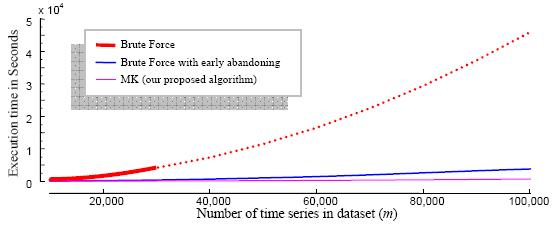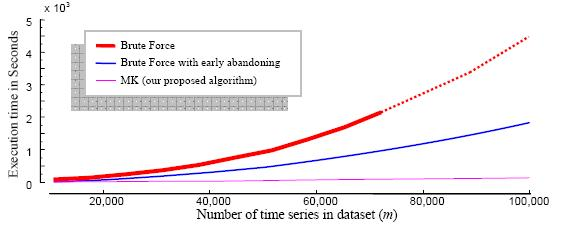Figure 6: The LSF5_10.txt is a time series of length 180,214 which is a sampled eeg data. The datasets for this experiment are sampled from the start of LSF5_10. All the execution times for the 10 runs on each of the 10 datasets in it can be found in the "speedup (real) details" sheet. Execution times for the Brute Force algorithms can be found in the "speedup (real) summary" sheet.
Why is Early-Abandoning so Effective?
Figure 7: We did this experiment on small samples from the LSF5 dataset. The samples can be found here. Statistics for this experiments (motif distances, average 1-NN distances and average distances) are found using BF_s code.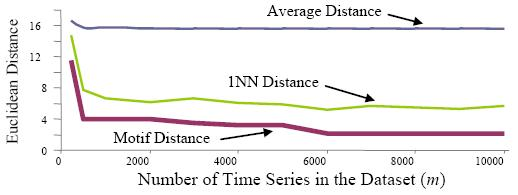Choosing the Number of Reference Points
Figure 8: We used this random_shuffle function to shuffle the 30,000 random walks from the Figure 5 dataset. We ran the mk_d.exe for 14 times on such shuffled data.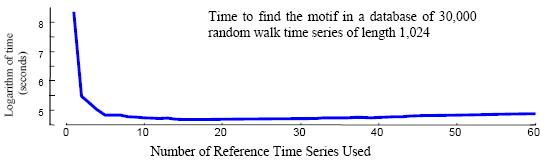Finding Repeated Insect Behaviors
Figure 14: The dataset is here. Its length is 33,021.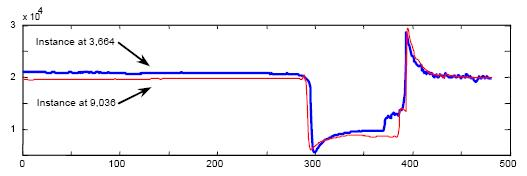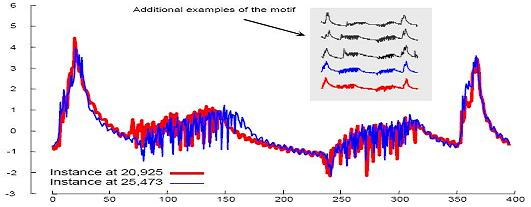Errata: The data set for Figure:15 is of length 18,667 and can be found here.
The entire trace containing the above dataset is of length 78,254 and can be found here.
Near Duplication Detection
The dataset we used have been taken from this page. We used the dataset of 1.5 million tiny images and use this code to generate 100,018 time series of length 768. These time series are created from the first 100,018 images in the DFS order. Since the data file is very large, we give the code only.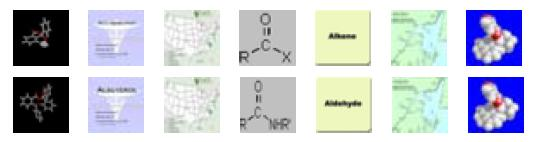Automatically constructing EEG Dictionaries
Figure 19 : The dataset for this experiment is LSF5_10.txt.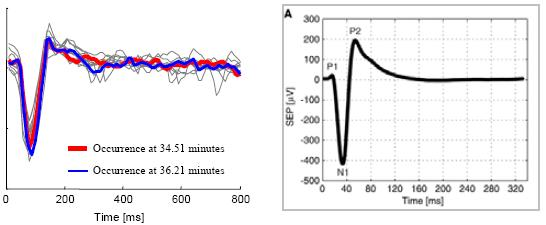Motif-Based Anytime Time Series Classification
Datasets can be found here.